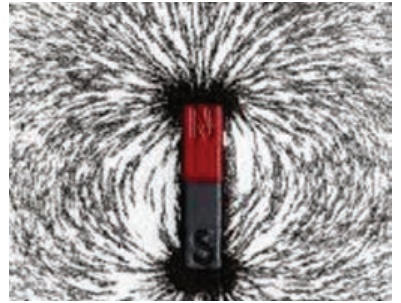Home | | Science 9th std | Magnetic Field Lines

# Magnetic Field Lines

A magnetic field line is de ned as a curve drawn in the magnetic field in such a way that the tangent to the curve at any point gives the direction of the magnetic field.

Magnetic Field Lines

Activity 2

Place a magnet over a cardboard. Sprinkle some iron-filings on the cardboard. Tap the card board gently. We observe that the iron filings align themselves in definite pattern. These patterns are called magnetic lines of force.Activity 2 shows that magnets have some curved lines around them and these lines are called magnetic field lines. is can also be inferred by placing a test magnet in the magnetic field of another magnet. rough the direction the test magnet moves, magnetic field lines can be identified. The magnetic field lines start at north pole and ends at south pole as shown in the Figure 3.4(a)

A magnetic field line is de ned as a curve drawn in the magnetic field in such a way that the tangent to the curve at any point gives the direction of the magnetic field as shown in the Figure 3.4 (b). In the Figure 3.4 (b), the arrow mark indicates the direction of magnetic field at points A, B and C. Note carefully that the magnetic field at a point is tangential to the magnetic Field lines.We all know that earth also behaves like a magnet and the magnetic field lines of earthâ€™s is shown in the figure 3.5## 1. Magnetic Flux

Magnetic flux is the number of magnetic field lines passing through a given area as shown in the figure 3.6. It is denoted by Ď• and its unit is weber (Wb).The number of magnetic field lines crossing unit area kept normal to the direction of field lines is called magnetic flux density. It is shown in the figure 3.7. Its unit is Wb/m2## 2. Properties of magneticlines of force

Â·           Magnetic lines of force are closed continuous curves, extending through the body of the magnet.

Â·           Magnetic lines of force start from the North Pole and end at the South Pole.

Â·           Magnetic lines of force never intersect.

Â·           They will be maximum at the poles than at the equator.

Â·           The tangent drawn at any point on the curved line gives the direction of magnetic field.

## 3. Magnetic field lines between two magnets

What does happen when two magnets are placed near each other? ere are four ways we can keep them. ey are shown in Fig 3.8(a) two unlike poles facing each other, (b) two like poles facing each other, (c) parallel magnets with same poles facing same side and (d) parallel magnets with opposite poles facing same side. All these positions are.

Study Material, Lecturing Notes, Assignment, Reference, Wiki description explanation, brief detail
9th Science : Magnetism and Electromagnetism : Magnetic Field Lines |